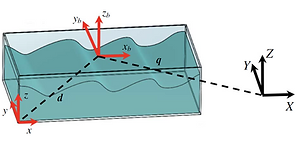top of page

# Dr Hamid Alemi Ardakani

###### Senior Lecturer in Mathematics

Department of Mathematics and Statistics

University of Exeter, Streatham Campus

Exeter EX4 4QF

ENGLAND## VARIATIONAL  PRINCIPLESVariational generalization of the Green–Naghdi and Whitham equations for fluid sloshing in three-dimensional rotating and translating coordinates

This paper derives an averaged Lagrangian functional for dynamic coupling between rigid-body motion and its interior shallow-water sloshing in three-dimensional rotating and translating coordinates; with a time-dependent rotation vector. A new set of variational shallow-water equations (SWEs) and generalized Green–Naghdi equations for the interior fluid sloshing with 3–D rotation vector and translations, and also the equations of motion for the linear momentum and angular momentum of the rigid-body containing shallow water, are derived from the averaged Lagrangian functional, which describes a columnar motion, by using Hamilton’s principle and the Euler–Poincaré variational framework. The generalized Green–Naghdi equations have a form of potential vorticity (PV) conservation, which can be obtained from the particle-relabeling symmetry, and is a combination of the PV derived by Miles and Salmon (1985) and the PV derived by Dellar & Salmon (2005) for geophysical fluid dynamics problems, where the rotation vector varies spatially. By applying the assumption of zero-potential-vorticity flow to the averaged Lagrangian functional, a new set of Boussinesq-like evolution equations are derived, which are a generalization of the Whitham equations for fluid sloshing in three-dimensional rotating and translating coordinates. Moreover, the new variational principles are appended to Luke’s variational principle to present a unified variational framework for the hydrodynamic problem of interactions between gravity-driven potential-flow water waves and a freely floating rigid-body, dynamically coupled to its interior weakly dispersive nonlinear shallow-water sloshing in three dimensions.

H. Alemi Ardakani 

Variational generalization of the Green–Naghdi and Whitham equations for fluid sloshing

in three-dimensional rotating and translating coordinates

European Journal of Mechanics B Fluids, 88: 208-227

H. Alemi Ardakani 

A variational principle for the generalized Whitham equations

(Preprint)

###### A variational principle for three-dimensional interactions between water waves and a floating rigid body with interior fluid motion

A variational principle is given for the motion of a rigid body dynamically coupled to its interior fluid sloshing in three-dimensional rotating and translating coordinates. The fluid is assumed to be inviscid and incompressible. The Euler–Poincaré reduction framework of rigid body dynamics is adapted to derive the coupled partial differential equations for the angular momentum and linear momentum of the rigid body and for the motion of the interior fluid relative to the body coordinate system attached to the moving rigid body. The variational principle is extended to the problem of interactions between gravity-driven potential flow water waves and a freely floating rigid body dynamically coupled to its interior fluid motion in three dimensions.H. Alemi Ardakani 

A variational principle for three-dimensional interactions between water waves and a floating rigid body with interior fluid motion

Journal of Fluid Mechanics866: 630-659

###### A variational principle for fluid sloshing with vorticity, dynamically coupled to vessel motion

A variational principle is derived for two-dimensional incompressible rotational fluid flow with a free surface in a moving vessel when both the vessel and fluid motion are to be determined. The fluid is represented by a stream function and the vessel motion is represented by a path in the planar Euclidean group. Novelties in the formulation include how the pressure boundary condition is treated, the introduction of a stream function into the EulerPoincar´e variations, the derivation of free surface variations, and how the equations for the vessel path in the Euclidean group, coupled to the fluid motion, are generated automatically.H. Alemi Ardakani, T.J. Bridges, F. Gay-Balmaz, Y. Huang, C. Tronci 

A variational principle for fluid sloshing with vorticity, dynamically coupled to vessel motion

Proceedings of the Royal Society of London A

## An alternative view on the Bateman-Luke variational principle

A new derivation of the Bernoulli equation for water waves in three-dimensional rotating and translating coordinate systems is given. An alternative view on the Bateman-Luke variational principle is presented. The variational principle recovers the boundary value problem governing the motion of potential water waves in a container undergoing prescribed rigid-body motion in three dimensions. A mathematical theory is presented for the problem of three-dimensional interactions between potential surface waves and a floating structure with interior potential fluid sloshing. The complete set of equations of motion for the exterior gravity-driven water waves, and the exact nonlinear hydrodynamic equations of motion for the linear momentum and angular momentum of the floating structure containing fluid, are derived from a second variational principle.H. Alemi Ardakani 

An alternative view on the Bateman-Luke variational principle

European Journal of Mechanics / B Fluids82: 39-46